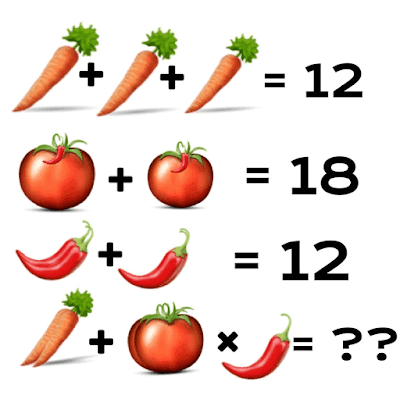### Maths Equation Puzzle! How Fast Can You Solve It

These interesting maths equations contain Mathematical variables which are represented by vegetables. Let's see how quickly you can solve it. Don't forget to explain how you arrived at your answer.#### Related Posts

###### MOUAU Student Fights Girlfriend in Front of Schoolmates Over ₦20K

4+9*6=78
Follow
Carrot= 12 / 3 = 4
Onion= 18/2 = 9
Pepper= 12 / 2 =6
4 + 9 x 6=
According to BODMAS
4 + (9 x 6)= ; 4 + 54
= 58
Follow
Carrot= 12/3=4
Tomato= 18/2=9
Pepper(in the the diagram is 2+1=3) therefore, 12/3=4
To the question
2carrots+1tomato*1pepper
1carrot=4, 2carrots= 4*2=8
1tomato= 9
1 pepper=4
8+9*4
8+(9*4) BODMAS
8+36=44
Follow
The answer is 62 how?
Carrot is 4 and we have two carrot thatis 8
Onion is 9

Pepper is 6
We have 8+9×6
BODMAS × before +
So 9×6=54
8+54=62 QED
Follow
Each carrot represents 4 digits; 4*3=12
Each tomatoes represents 9 digits;9*2=18
Each pepper represnts 3 digits and there are 2pairs of peper i.e(3*2)+(3*2)=12
If you check the tomatoes well,it has 1pepper each on it....therefore its the addition of the pepper and tomatoes that gives 9 and since we now now that the pepper is 3,then Tomatoes;9-3=6. i.e Each tomato represents 6
Now the final answer goes thus;
2 carrots + 2 tomatoes ×1 pepper .......
(4×2) +(2×6) ×(1×3)=8+12×3
According to bodmas=8+(12×3)
8+36=44
Therefore 44 is the final answer!!!!!
Follow
78
Follow

#### Quick Questions

• 0 Answers   ·   5 minutes ago
• 1 Answers   ·   3 hours ago
• 3 Answers   ·   17 hours ago

#### Post Comment

Please don't post or ask to join a "Group" or "Whatsapp Group" as a comment. It will be deleted. To join or start a group, please click here

11 Members
5 Members
• 26th
Mar 2019
• 26th
Mar 2019
##### Marketplace
• Here are the things students around you have to sell;
• ###### Integrated Points on Government for UTME students
₦700   OAU   New
• ###### 27 front-end and back-end (full-stack) Highly rated video tutorials
₦10,000   UNILAG   New